## Saturday, February 23, 2013

### Greetings From Riverside! Mike Grigsby's Blog and an TI nSpire App ReviewI am blogging today from Daily Brew Coffee House in Riverside, CA. Service here is excellent - very nice and friendly people.

Mike Grigsby

If you get a chance, please check out Mike Grigsby's blog at http://mikebibbygrigsby83.blogspot.com/?m=1. Mike has a Master's Degree in Mathematics from Cal Poly Pomona, and is the Social Media Administrator for the Riverside Astronomical Society. Later tonight I am going to meet with Mike to go their monthly meeting at La Sierra University in Riverside.

Mike is a super duper awesome guy. Please check his blog out.

Quick Review: Texas Instruments TI-nSpire App for iPad

Cost: \$29.99 (yes, this is very expensive for an app). There is a CAS and non-CAS version. I totally recommend that you get the CAS version. (CAS is the Computer Algebraic Version)
Version of nSpire: TI nSpire CX (the latest and the good one in my opinion)

Short and sweet, the nSpire app is everything the TI nSpire hardware is. Unlike most calculators, the nSpire operates as a computer software interface (think closer to Mathematica, Matlab, or Maple) than the traditional calculator. You work on documents which contains pages. Each page can be a mathematics page, graph page, a spreadsheet, geometry page, or a statistics page. Within the problems in the document, variables are linked. That is, you can create a variable in one page, and it is define throughout the documents. Programs work the same way.

Programs are in TI-Basic and can either be limited to the document or made global. The global feature is very useful when you are creating custom functions (like the gamma function). Programming on the nSpire is more strictly for the mathematical sense; so if you are looking to make video games, this app may not be what you are looking for.

The keyboard on the app is a pleasure to use. On the keyboard, you will note several keys having a blue bar over them. Holding the key down gives you additional options. For example:
The var key contains the STO> function.
The sin key expands to sin^-1, csc, and csc^-1.

The best part of the keyboard? The keys are big! Well, we are on an iPad here.

On the graph page, not only can you graph functions, polar functions, scatter plots, parametric functions, and inequalities, you can do it all one page if you want. This adds flexibility that is usually seen only on the Casio graphing calculators and somewhat on the HP 49g series. The graph page offers a geometric view or a 3D view for three-dimensional graphs. Pinch to zoom, hold on to drag the graph and any other text.

Here are the screenshots from the app. All the shots are from the CAS version.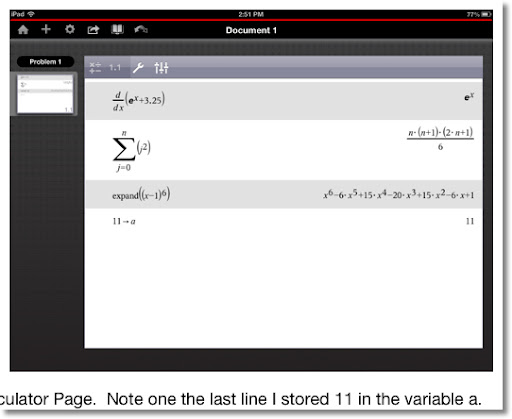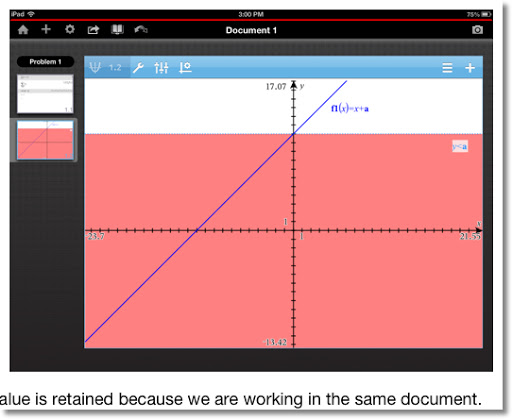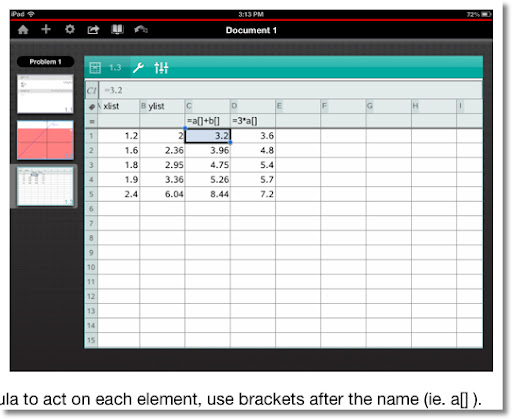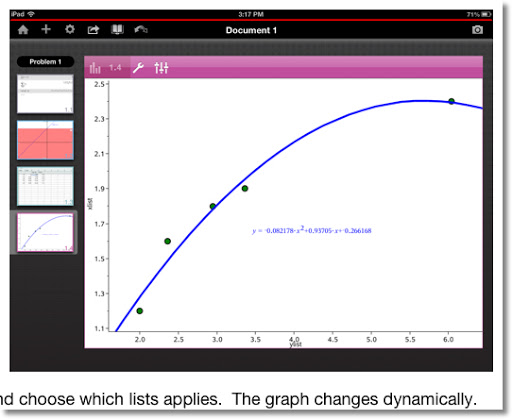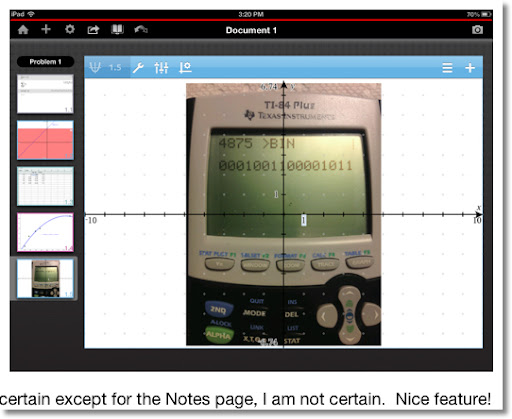I recommend getting this app. Now I realize that the \$29.99 price tag is heavy in the market on apps, but this app has it all together in one package. However, in the market of complete mathematical software packages it is inexpensive. If you buy it, I recommend the CAS version.

This is also a great alternative to getting the hardware if you don't want and/or have \$150 to spend but have an iPad ready. And the batteries last longer! At least with the app I don't have to constantly plug in my tangible nSpire CX almost every time I want to use it. And yes documents can be shared by email or downloaded to the computer for later use (including downloading it on the physical units).

That is all for today, please go check out Mike Grigsby's blog. I can't wait to meet with him tonight. Have a great day and weekend everyone.

Eddie

This blog is property of Edward Shore. 2013

## Wednesday, February 20, 2013

### TI-84+: Binary-Decimal Conversions

One of the missing features of the TI-82/83/84 family is the ability to convert between bases. Here are two programs in TI-Basic to help fill at least some of the gap.

It is very basic conversion, working only with positive integers up to 65,535 (16 ones as its binary representation).

Variables used:
N = number in decimal form
L1 = list representing the binary representation (1s and 0s)

The programs display the binary numbers as a solid number, rather by a list. This is accomplished by a For loop involving the Output command.

Access L1 by pressing [2nd], [ 1 ].

Examples: Decimal ← → Binary
27 ← → {0,0,0,0,0,0,0,0,0,0,0,1,1,0,1,1}
428 ← → {0,0,0,0,0,0,0,1,1,0,1,0,1,1,0,0}
3,245 ← → {0,0,0,0,1,1,0,0,1,0,1,0,1,1,0,1}

DEC2BIN
Decimal to Binary (N → L1)
This program works with any positive integer from 0 to 65,535 - 16 bits. No negative numbers. Note: ending quotes and parenthesis are left out to conserve space.
2/20/2013. 170 bytes.  (updated 7/5/2016)

: Input "N:",N
: If N<0

: Then
: Pause "INVALID
: Stop
: End
: int(N→N
: N→D
: DelVar L1
: 16→dim(L1
: For(K,0,15
: If 2^(15-K)≤D
: Then
: 1→L1(K+1
: D-2^(15-K→D
: End
: End
: ClrHome
: Output(1,1,N
: Output(1,6,">BIN
: For(K,1,16
: Output(3,K,L1(K
: End
: Pause
: ClrHome

BIN2DEC
Binary to Decimal (L1 → N). Enter a list up to 16 zeroes and ones.
This program works with any positive integer from 0 to 65,535 - 16 bits. No negative numbers. Note: ending quotes and parenthesis are left out to conserve space.
2/20/2013. 160 bytes.

: Input "L1 UP TO 16 BITS:",L1
: If dim(L1)>16
: Then
: Pause "INVALID
: Stop
: End
: While dim(L1) < 16

: augment({0},L1→L1
: End
: 0→N
: For(K,0,15
: N+2^K*L1(16-K→N
: End
: ClrHome
: For(K,1,16
: Output(1,K,L1(K
: End
: Output(3,1,">DEC
: Output(3,6,N
: Pause
: ClrHome

** Edited 12/5/2013.  This is due to an error Stephanie Ison pointed out to me.  Many thanks! - Eddie

Enjoy!

Eddie

This blog is property of Edward Shore. 2013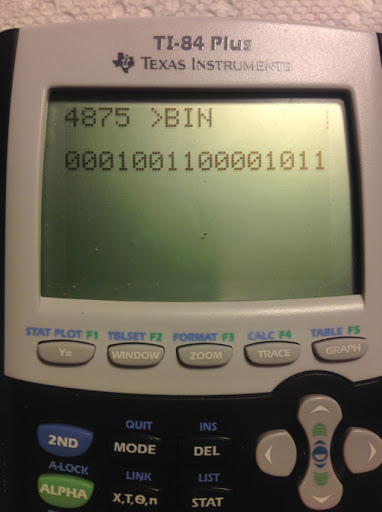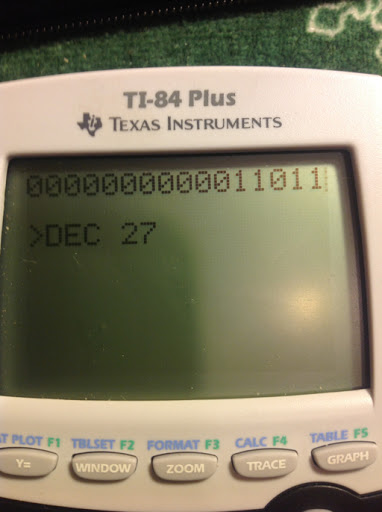## Saturday, February 16, 2013

### Review: Programming the TI-83 Plus/TI-84 Plus by Christopher R. Mitchell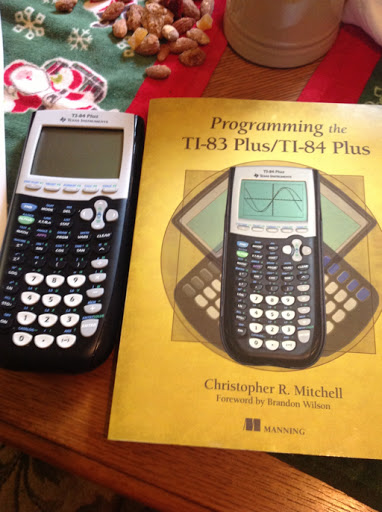Continuing the Saturday night tour, I stopped by at another coffee place, the Swörk Urban Coffee Bar in Eagle Rock (Los Angeles).

Review: Programming the TI-83 Plus/TI-84 Plus - Christopher R. Mitchell

Author: Christopher R. Mitchell, his website is http://www.cemetech.net/.

To make this short and sweet, this book is an excellent reference book, for all levels. I have 22 years of calculator programming experience - starting from the TI-81 in 1991. However I am learning new tricks and techniques from this book.

The book aims towards the TI-83/TI-84 family and how to maximize programming efficiency. I focused on the later chapters of the book, where topics cover the GETKEY command, using the home and graph screen to efficiently display text and figures, and how to make programs interactive. There is also a chapter on how to optimize programs, such as taking advantage of implicit conditionals and use of the last answer (Ans) function. So far, the GETKEY is my favorite thing I am learning.

The list price is \$29.99, which includes the book and electronic copies of the book (I have yet to download the electronic copy.) If you want to expand your programming skills, or develop them from the beginning - Go for it, I definitely recommend this book. Mitchell writes the book in a straight-forward manner.

P.S. I got to the mouse to eat 142 pieces of cheese. (See chapter 6 to see what I am taking about, readers).

Eddie

This blog is property of Edward Shore. 2013### a_(n+1) = a_n + n + C (from Stories Cafè in Echo Park in Los Angeles)

Good afternoon all! This is a Saturday Afternoon/Night blog post. On the menu: much needed delicious food from Stories Cafè in Los Angeles and some work with recurrence relations.

But first, an announcement! I am working on a short series of programming tutorials of the Hewlett Packard HP 39gii Calculator. Think of it as a short boot camp to get HP 39gii owners up and running.

An emulator for the HP 39gii can be found here: http://www.hp.com/sbso/product/calculators-emulators/graphic-calculator-emulators.html. The emulators are for Widows and probably Mac - not sure if they run on other operating systems. As of today, I am not aware if there are any iOS and/or Android apps for this. I aim to post this series in March 2013.Now on to the math...

a_(n+1) = a_n + n + C

The goal is to find a general formula for

(I) a_(n+1) = a_n + n + C with the initial condition a_0 = A_0.

The type of recursion formula presented is polynomial recurring relation. A general polynomial recursion formula takes the form a_(n+1) = a_n + p(n).

The general formula for a_(n+1) = a_n + n + C takes the form:

(II) a_n = c_2 * n^2 + c_1 * n + c_0

Mainly, with recursion formula of polynomial type will require a formula of order n+1. There are n+2 constants to solve for.

Working with equation (II) above, we will need to solve for c_0, c_1, and c_2. It would be very difficult to work with only one equation. Luckily, we can turn this problem in to a system of three linear equations.

Starting with the initial condition a_0 = A_1, we can use the recursion formula for n=1 and n=2 to find a_1 and a_2. Then we get the system:

(III)
a_0 = c_0 (where n=0)
a_1 = c_0 + c_1 + c_2 (where n=1)
a_2 = c_0 + 2 * c_1 + 4 * c_2 (where n=2)

Putting (III) in matrix form we get:

(IV)
[ [1, 0, 0], [1, 1, 1], [1, 2, 4] ] * [ [c_0], [c_1], [c_2] ] = [ [a_0], [a_1], [a_2] ]

For the Hewlett Packard HP 50g calculator owners (others may have this function/program), you can get the matrix on the left hand side of equation (IV) by using the VANDERMONDE matrix function on the vector [0, 1, 2]. This function comes in handy for these types of problems.

Continuing with the ever handy HP 50g, solving for c_0, c_1, and c_2:

(V)
[ [c_0],[c_1],[c_2] ] = [ [1, 0, 0],[-3/2, 1, -1/2],[1/2, -1, 1/2] ] * [ [a_0], [a_1], [a_2] ]

And we finish with:
(VI)
c_0 = a_0
c_1 = -3/2 * a_0 + 2 * a_1 - 1/2 * a_2
c_2 = 1/2 * a_0 - a_1 + 1/2 * a_2

So in summary:
The general formula for the recursion formula

a_(n+1) = a_n + n + C is:

c_0 = a_0
c_1 = -3/2 * a_0 + 2 * a_1 - 1/2 * a_2
c_2 = 1/2 * a_0 - a_1 + 1/2 * a_2

A numerical example:

a_(n+1) = a_n + n - 2 with a_0=3

Prepare by finding a_0, a_1, and a_2:

a_0 = 3 (given)
a_1 = 3 + 1 - 2 = 2 (use n=1)
a_2 = 2 + 2 - 2 = 2 (use n=2)

Then:
c_0 = 3
c_1 = -3/2 * 2 + 2 * 2 - 1/2 * 2 = -3/2
c_2 = 1/2 * 2 - 2 + 1/2 * 2 = 1/2

Our general formula is:
a_n = 1/2 * n^2 - 3/2 * n + 3

Let's test it out.

With n=1:
a_1 = 1/2 - 3/2 + 3 = 2 (checks out)

With n=3
a_3 = 1/2 * 3^2 - 3/2 * 3 + 3 = 3

Observe from the recursion formula a_3 = 2 + 3 - 2 = 3. (Checks out)

With n=4
a_4 = 1/2 * 4^2 - 3/2 * 4 + 3 = 5

Warning: The above technique only works when the coefficient of a_n is 1. (See (I).)

I tried this technique with a_(n+1) = -3*a_n + 7*n - 1 with a_0 =1.

With a_1 = 5 and a_2 = 0 from the recursion formula, I came up with a_n = -9/2 * n^2 + 17/2 * n + 1. Using this formula I came up with a_3 = -14, when in reality a_3 = 22. Just a heads up.

I hope this weekend and all your future days are good to you.

Eddie

This blog is property of Edward Shore. 2013

## Saturday, February 9, 2013

### a_(n+1) = S * a_n + T

Hi everyone!

First a correction. In my Numerical CAS section, posted December 2012, I typed an extra quotation mark in the POLYBINE program for the Casio Prizm - that has been corrected. Much thanks to Ryan Maziarz who pointed that out to me.

Here is a link to the corrected post:
http://edspi31415.blogspot.com/2012/12/numeric-cas-part-2-binomial-expansion.html?m=1

Today we are finding the general formula for this recursion formula:

a_(n+1) = S * a_n + T

with the initial condition a_0 = A.

I could try using a characteristic polynomial, but with some observation we may be able to detected a pattern which leads us to a general formula.

Observe that:

a_0 = A

a_1 = S * a_0 + T
a_1 = S * A + T

a_2 = S * a_1 + T
a_2 = S * (S * A + T) + T
a_2 = S^2 * A + S * T + T
a_2 = S^2 * A + T * (S + 1)

a_3 = S * a_2 + T
a_3 = S * (S^2 * A + T * (S + 1)) + T
a_3 = S * A^3 + T * (S^2 + S + 1)

a_4 = S * a_3 + T
a_4 = S^4 * A + T * (S^3 + S^2 + S + 1)

From the pattern, observe that:

a_n = S^n * A + T * Σ(S^k, k=0, n-1)

With Σ(t^k, k=0, n-1) = (t^n - 1)/(t - 1) :

a_n = S^n * A + T * (S^n - S)/(S - 1)

An Example:

S = 3, T = 1, A = -2

Using the HP 39gii to generate the sequence (the 39gii uses a_1 as its initial term):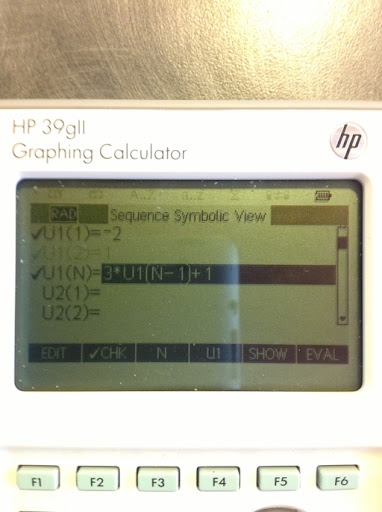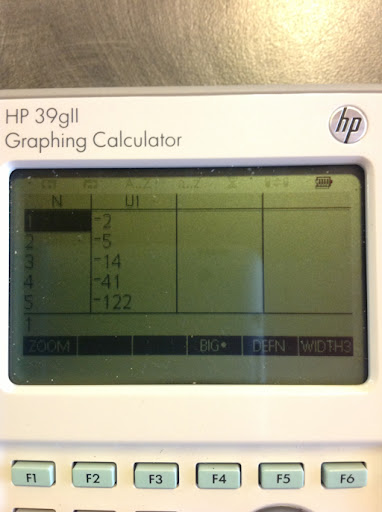The general formula is:

a_n = (3^n) * (-2) + 1 * (3^n - 1)/(3 - 1)
a_n = (-2) * 3^n + (3^n - 1)/2
with a_0 = -2. (The 39gii has u_1 = a_0)

Example:

a_2 = u_3 = (3^2) * (-2) + (9 - 1)/2 = -14

That is all for now, see you next time!

Eddie

This blog is property of Edward Shore. 2013

5 blog entries away from the 200th blog entry.

### Greetings From Monrovia! Finding a Formula to Generate the Fibonacci SequenceHi everyone. I am blogging from the Friends Café in downtown Monrovia, CA on a beautiful, chilly day.

Today's blog entry is about to the Fibonacci Sequence and how to find a general formula to generate a sequence.

The Fibonacci Sequence

The world famous Fibonacci Sequence, first introduced by Fibonacci's Liber Abaci, written in 1202, is:

1, 1, 2, 3, 5, 8, 13, 21, 34, 55, 89, 144, 293, 377...

(Source: Pickover, Clifford. "The Math Book" Sterling Publishing, New York. 2009 - Eddie says: Go get this book - it's awesome!)

It is easy to build the sequence. Start with two entries of 1. Add them up to get the next term. Each subsequent term is the sum of the last two. 1+1=2, 1+2=3, 2+3=5, 3+5=8, 5+8=13, and so on.

We can use this recursion formula:

a_(n+2) = a_(n+1) + a_n

with the initial conditions a_0 = 1 and a_1 = 1.

We can generate a general formula for this.

Technique

a_(n+2) = a_(n+1) + a_n

with the initial conditions a_0 = 1 and a_1 = 1.

This is a linear recursion formula. A way to find the general formula is to use a characteristic polynomial. The characteristic polynomial is formed by turning subscripts to exponents (and usually replacing a with another letter, such as x) and finding the roots of the polynomial.

The general formula will have the form

a_n = c_1 * (x_1)^n + c_2 * (x_2)^n + ...

Where n is the number of initial conditions.

In the case of the Fibonacci sequence, we have two initial conditions (a_0 = 1 and a_1 = 1). Hence n=2.

Then use the initial conditions to solve for the c_k constants.

Finding the General Formula

a_(n+2) = a_(n+1) + a_n
with the initial conditions a_0 = 1 and a_1 = 1.

The characteristic equation is:

x^(n+2) = x^(n+1) + x^n

Assuming x ≠ 0, divide both sides by x^n:

x^2 = x + 1

x^2 - x - 1 = 0

The roots of the polynomial are:

x_1 = (1 + √5)/2
x_2 = (1 - √5)/2

Then our general form has:

a_n = c_1 * ((1 + √5)/2)^n + c_2 * ((1 - √5)/2)^n

Our next step is to find c_1 and c_2.

Note when n = 0, a_0 = 1 and:

1 = c_1 + c_2

When n = 1, a_1 = 1 and:

1 = c_1 * (1 + √5)/2 + c_2 * (1 - √5)/2

Leaving us with the system of linear equations:

c_1 + c_2 = 1
c_1 * (1 + √5)/2 + c_2 * (1 - √5)/2 = 1

I like using matrices to solve systems of linear equations, you may a different preferred way, it's all good here:

[ [1, 1], [(1 + √5)/2, (1 - √5)/2] ] * [ [c_1], [c_2] ] = [ [ 1 ], [ 1 ] ]

[ [c_1], [c_2] ] = [ [1, 1], [(1 + √5)/2, (1 - √5)/2] ]^-1 * [ [ 1 ], [ 1 ] ]

[ [c_1], [c_2] ] = [ [(5 - √5)/10, √5/5], [(5 + √5)/10, -√5/5] ] * [ [ 1 ], [ 1 ] ]

which evaluates and simplifies to:

c_1 = (5 + √5)/10
c_2 = (5 - √5)/10

The general formula to generate the Fibonacci sequence is:

a_n = (5 + √5)/10 * ((1 + √5)/2)^n + (5 - √5)/10 * ((1 - √5)/2)^n

An approximate formula would be (8 digits):

a_n ≈ 0.72360680 * 1.6180339^n + 0.27639320 * (-0.6180339)^n

Round off when necessary.

Testing the general formula, find the 2nd and 6th term:

a_2 = (5 + √5)/10 * ((1 + √5)/2)^2 + (5 - √5)/10 * ((1 - √5)/2)^2
a_2 = (5 + √5)/5 + (5 - √5)/2
a_2 = 10/2 = 2

a_6 = (5 + √5)/10 * ((1 + √5)/2)^6 + (5 - √5)/10 * ((1 - √5)/2)^6
a_6 = (65 + 29 * √5)/10 + (65 - 29 * √5)/10
a_6 = 130/10 = 13

The technique applied can be applied to the general problem:

a_(n+2) = S * a_(n+1) + T * a_n

With initial conditions a_0 = A and a_1 = B.

Using the trusty HP 50g to assist me, I come up with the following:

a_n = c_1 * (x_1)^n + c_2 * (x_2)^n

where

x_1 = (S + √(S^2 + 4 * T))/2

x_2 = (S - √(S^2 + 4 * T))/2

c_1 = (B - A * x_2)/(x_1 - x_2)

c_2 = (A * x_1 - B)/(x_1 - x_2)

Next time, I am going to look at the general recursion formula:

a_(n+1) = S * a_n + T with the initial condition a_0 = A

I am working a basic programming series, which my target is with the HP 39gii calculator and the series would be posted in March 2013.

Thank you everyone who reads, follows, and comments on this blog. As always it is much appreciated.

Have a great day!

Eddie

This blog is property of Edward Shore. 2013

## Friday, February 1, 2013

### Balances and Recursion Formula - Part 2

To recap our scenario:

We have a loan with an initial balance \$b, that has an annual interest rate of r%. We are making a monthly payment of \$p every month. In Part 1, we were finding out how long to pay off the balance.

In Part 2, we asking the same question, but with an added component: this time assume that there is a monthly charge of \$c to the balance. The charge stops when the balance is paid.

Variables:
b = a_0 = initial balance
p = monthly payment
r = annual rate
a_n = balance after n payments

Let the recursion formula be:

a_(n+1) = (a_n + c) + (a_n + c) × r/1200 - p
with the initial condition a_0 = b.

Let θ = r/1200, then:
a_(n+1) = (a_n + c) × (1 + θ) - p

Let's get a_1, a_2, and a_3 in terms of a_0.

a_1 = (a_0 + c) × (1 + θ) - p

a_2 = (a_1 + c) × (1 + θ) - p
a_2 = ((a_0 + c) × (1 + θ) - p + c) × (1 + θ) - p
a_2 = (a_0 + c) × (1 + θ)^2 - p × (1 + θ) + c × (1 + θ) - p

a_3 = (a_2 + c) × (1 + θ) - p
a_3 = a_2 × (1 + θ) + c × (1 + θ) - p
a_3 = ((a_0 + c) × (1 + θ)^2 - p × (1 + θ) + c × (1 + θ) - p) × (1 + θ) + c × (1 + θ) - p
a_3 = (a_0 + c) × (1 + θ)^3 - p × (1 + θ)^2 + c × (1 + θ)^2 - p × (1 + θ) + c × (1 + θ) - p

Noticing a pattern...

a_n = (a_0 + c) × (1 + θ)^n - p × Σ((1 + θ)^k, k=0, n-1) + c × Σ((1 + θ)^k, k=1, n-1)
a_n = (a_0 + c) × (1 + θ)^n - p × ((1 + θ)^n - 1)/θ + c × ((1 + θ)^n - (1 + θ))/θ

With a_0 = b:

a_n = (b + c) × (1 + θ)^n - p × ((1 + θ)^n - 1)/θ + c × ((1 + θ)^n - (1 + θ))/θ

Example 1:

Sandra has a credit card with a 15% APR. The initial balance is \$1,500.00. Sandra uses a premium credit card that charges \$20.00 each month there is a balance.

b = 1500.00
c = 20.00
r = 15%
p = 500.00

Then θ = 15/1200 = 1/80

The resulting sequence is:

a_0 = 1500.00
a_1 = 1039.00
a_2 = 572.23
a_3 = 99.64

In 3 payments , Sandra has knocked the balance to below the payment amount of \$1,500.00. In month 4, the amount will be (\$99.64 + \$20.00) × (1 + 15/1200) = \$121.14, and her debt will be over.

Example 2:
Greg has taken a payday loan, for the amount of \$1,893.64. The interest rate is 14.99% and the loan incurs a \$14.99 holding fee for each month the balance exists. Greg makes a \$350.00 payment every month.

b = 1893.64
c = 14.99
r = 15.99%
p = 350.00

Then θ = 15.99/1200

The sequence generated is:

a_0 = 1893.64
a_1 = 1584.06
a_2 = 1270.36
a_3 = 952.48
a_4 = 630.36
a_5 = 303.95

At month 6, the final balance will be (\$303.95 + \$14.99) × (1 + 14.99/1200) = \$322.92.

We can find out when the balance is zero by solving for n.

When a_n = 0:

n = ln((c × (1 + θ)/θ - p/θ) / (b + c - p/θ +c/θ)) × (ln (1 + θ))^(-1)

Then the integer part of n, int(n), is the nth payment when the balance is less than the payment amount.

Recalling our examples:

Example 1:

b = 1500.00
c = 20.00
r = 15%
p = 500.00

Then θ = 15/1200 = 1/80, and n ≈ 3.21

This means the balance is below the payment after then 3rd payment.

Example 2:

b = 1893.64
c = 14.99
r = 15.99%
p = 350.00

Then θ = 15.99/1200 and n ≈ 5.91

The balance becomes below the payment after the 5th payment.

Have a great day everyone!

Eddie

This blog is property of Edward Shore. 2013

### Balances and Recursion Formula - Part 1Greetings everyone coming to you from a Starbucks in Covina, CA! (on my way to seeing my mom and some friends for dinner).

1 month of 2013 is already in the books - hope everyone is getting February off to a great start!

The topic of this and the next post: using a recursion formula in financing.

The scenario: You have a loan that you make monthly payments. Interest accrues each month based on the loan's balance. The rate is stated as an annual rate. How many months will it take to pay off the loan?

For this, we are going to use a recursion formula to describe the balance at the end of each payment. We will then come up with a general formula which will allow us to find the balance at any month. Finally, use the general formula to find when the balance is zero (approximately).

Part 1 will deal with the scenario stated. On my next blog entry, I will consider paying the loan balance when a monthly charge is involved.

What is a recursion formula?

A recursion formula is a formula in which the result is based on previous results. To generate a sequence of answers, one or more initial conditions are required.

A simple example is:

a_(n+1) = 2 × a_n + 1 with the initial condition a_0 = 0.

In order to calculate a_(n+1), you will need the previous result, a_n.

Then: (a_n is in bold)

a_1 = 2 × 0 + 1 = 1
a_2 = 2 × 1 + 1 = 3
a_3 = 2 × 3 + 1 = 7
a_4 = 2 × 7 + 1 = 15
And so on...

Some recursion formulas can be transformed into general formulas, where any nth term can be found when only the initial conditions are known.

A famous recursion formula is the Fibonacci Sequence (1, 1, 2, 3, 5, 8, 13, 21, etc...)

a_(n+2) = a_(n+1) + a_n with the initial conditions a_0 = 1 and a_1 =1.

Back to our scenario: You have a loan that you make monthly payments. Interest accrues each month based on the loan's balance. The rate is stated as an annual rate. How many months will it take to pay off the loan?

Variables:
b = a_0 = initial balance
p = monthly payment
r = annual rate (if the rate is 10%, r = 10)
a_n = balance after n payments (after n months)

Let the recursion formula be:
a_(n+1) = a_n + a_n × r/1200 - p
with the initial condition a_0 = b

Let θ = r/1200. Then:

a_(n+1) = a_n × (1 + θ) - p

Note: we will stop when the balance sinks below the payment amount. At that point, the next payment is the balance, reducing the debt to 0.

Calculating the first few terms (balance after n payments) in terms of the initial balance (a_0):

a_1 = a_0 × (1 + θ) - p

a_2 = a_1 × (1 + θ) - p
a_2 = (a_0 × (1 + θ) - p) - p
a_2 = a_0 × (1 + θ)^2 - p × (1 + θ) - p

a_3 = a_2 × (1 + θ) - p
a_3 = (a_0 × (1 + θ)^2 - p × (1 + θ) - p) × (1 + θ) - p
a_3 = a_0 × (1 + θ)^3 - p × (1 + θ)^2 - p × (1 + θ) - p

Noticing the pattern...

a_n = a_0 × (1 + θ)^n - Σ( p × (1 + θ)^k, k=0, n-1)
a_n = a_0 × (1 + θ)^n - p × Σ( (1 + θ)^k, k=0, n-1)
a_n = a_0 × (1 + θ)^n - p × ((1 + θ)^n - 1)/(1 + θ - 1))

With a_0 = b:

a_n = b × (1 + θ)^n - p × ((1 + θ)^n - 1)/θ

Example 1:

A bank loan is made for \$1,000, which has a 10% annual interest rate. The borrower plans to make monthly payments of \$300.

r = 10%, b = 1000, p = 300
Then θ = 10/1200 = 1/120

The sequence generated is:

a_0 = 1000.00
a_1 = 708.33
a_2 = 414.23
a_3 = 117.68

Example 2:

The balance on a short term lease is currently \$8,764.10. The lessor wants to make payments of \$1,500 until the balance is fully paid. The lease carries an interest rate of 4.95%.

r = 4.95%, b = 8764.10, p = 1500.00
Then θ = 4.95/1200 = 33/8800

The sequence generated is:

a_0 = 8764.10
a_1 = 7300.25
a_2 = 5830.37
a_3 = 4354.42
a_4 = 2872.38
a_5 = 1384.27

When will the balance be fully paid?

Solving for n when a_n = 0:

0 = b × (1 + θ)^n - p × ((1 + θ)^n - 1)/θ
0 = (b - p/θ) × (1 + θ)^n + p/θ
-p/θ = (b - p/θ) × (1 + θ)^n
-[ (p/θ) / (b - p/θ) ] = (1 + θ)^n
[ (p/θ) / (p/θ - b) ] = (1 + θ)^n
ln [ (p/θ) / (p/θ - b) ] = n × ln (1 + θ)

n = ln [ (p/θ) / (p/θ - b) ] / ln (1 + θ)

Taking the integer portion of n, or int(n), gives the nth payment when the balance is less than the payment amount.

Continuing with our two examples from before:

Example 1:

r = 10%, b = 1000, p = 300
Then θ = 10/1200 = 1/120

n ≈ 3.3

After 3 payments, the balance is below the payment amount of \$1,000.00.

Example 2:

r = 4.95%, b = 8764.10, p = 1500.00
Then θ = 4.95/1200 = 33/8800

n ≈ 5.9

After 5 payments, the balance is below the payment amount of \$1,500.00.

Next time will be Part 2. Thanks as always!

Eddie

This blog is property of Edward Shore. 2013

P.S. To all those who are partaking in the Super Bowl festivities this Sunday: please be safe - and don't drink and drive! And have fun.

### HP 15C: Error Function and Lower Tail Normal Cumulative Function

HP 15C:   Error Function and Lower Tail Normal Cumulative Function Formulas Used  Error Function  erf(x) = 2 ÷ √π * ∫( e^(-t^2) dt, t = 0 t...